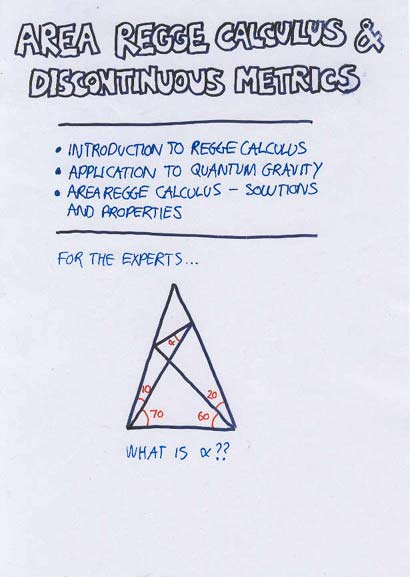# Maths and Physics > My Work

## My Publications

• A. F. Kleppe and Chris Wainwright, Super coset space geometry, J. Math. Phys. 48 (2007) 053511, hep-th/0610039

Super coset spaces play an important role in the formulation of supersymmetric theories. The aim of this paper is to review and discuss the geometry of super coset spaces with particular focus on the way the geometrical structures of the super coset space G/H are inherited from the super Lie group G. The isometries of the super coset space are discussed and a definition of Killing supervectors - the supervectors associated with infinitesimal isometries - is given that can be easily extended to spaces other than coset spaces.

• A. F. Kleppe and Chris Wainwright, Graded Majorana spinors, J. Phys. A: Math. Gen. 39 (2006) 3787-3799, hep-th/0501252

In many mathematical and physical contexts spinors are treated as Grassmann odd valued fields. We show that it is possible to extend the classification of reality conditions on such spinors by a new type of Majorana condition. In order to define this graded Majorana condition we make use of pseudo-conjugation, a rather unfamiliar extension of complex conjugation to supernumbers. Like the symplectic Majorana condition, the graded Majorana condition may be imposed, for example, in spacetimes in which the standard Majorana condition is inconsistent. However, in contrast to the symplectic condition, which requires duplicating the number of spinor fields, the graded condition can be imposed on a single Dirac spinor. We illustrate how graded Majorana spinors can be applied to supersymmetry by constructing a globally supersymmetric field theory in three-dimensional Euclidean space, an example of a spacetime where standard Majorana spinors do not exist.

• A. F. Schunck and Chris Wainwright, A geometric approach to scalar field theories on the supersphere, J. Math. Phys. 46 (2005) 033511, hep-th/0409257

Following a strictly geometric approach we construct globally supersymmetric scalar field theories on the supersphere, defined as the quotient space S^{2|2} = UOSp(1|2)/U(1). We analyze the superspace geometry of the supersphere, in particular deriving the invariant vielbein and spin connection from a generalization of the left-invariant Maurer-Cartan form for Lie groups. Using this information we proceed to construct a superscalar field action on S^{2|2}, which can be decomposed in terms of the component fields, yielding a supersymmetric action on the ordinary two-sphere. We are able to derive Lagrange equations and Noether's theorem for the superscalar field itself.

• Chris Wainwright and Ruth M. Williams, Area Regge calculus and discontinuous metrics, Class. Quant. Grav. 21 (2004) 4865-4880, gr-qc/0405031

Taking the triangle areas as independent variables in the theory of Regge calculus can lead to ambiguities in the edge lengths, which can be interpreted as discontinuities in the metric. We construct solutions to area Regge calculus using a triangulated lattice and find that on a spacelike hypersurface no such discontinuity can arise. On a null hypersurface however, we can have such a situation and the resulting metric can be interpreted as a so-called refractive wave.

## Talks

• Area Regge calculus and discontinuous metrics